# Division by Sharing Equally

Division by Sharing Equally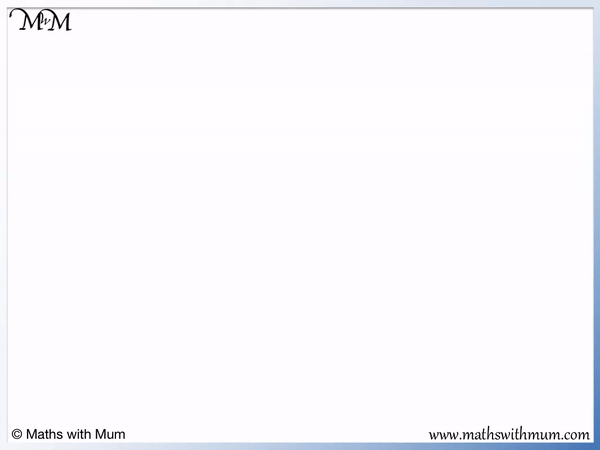• Division means to share an amount equally.
• We will share 6 counters so that there is the same number of counters in each of the 3 pots.
• We place one counter in each of the pots.
• We then place another counter in each of the pots.
• There are no more counters left and each pot has the same number of counters inside it.
• There are 2 counters in each of the 3 pots.
• 6 shared equally between 3 is 2.
• We can say that 6 divided by 3 equals 2.
• We can write this as 6 ÷ 3 = 2.
Division means to share an amount equally.• We can use sharing equally to work out 10 ÷ 2.
• We first draw circles as the 2 groups.
• We will add one counter at a time to each of the 2 groups, keeping the same amount in each group.
• We can put 5 counters in each of the 2 groups.
• 10 shared equally into 2 groups gives us 5 in each group.
• We say that 10 ÷ 2 = 5.# Division by Sharing Equally

## What is Division by Sharing?

Division by sharing equally is a method used to introduce the concept of division. It involves sharing an amount equally between groups one at a time until there is the same amount in each group. Division by sharing is often taught using physical objects such as counters.

Division by sharing equally is the best method for introducing the idea division. This is because it only involves simple counting skills and the sharing method lends itself to physical objects such as counters, which children enjoy using.

We can think of division as sharing.

The number before the division sign is the total amount being shared. The number after the division sign is the number of groups that the total is being shared between.

The answer to the division is the amount that is shared into each group.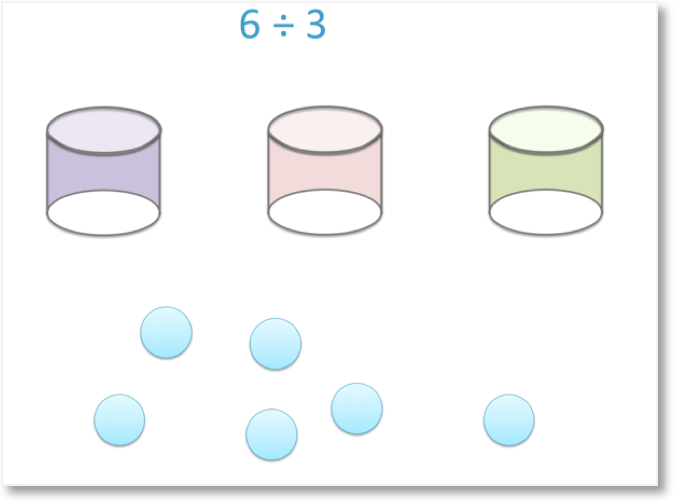For example here is the division question of 6 ÷ 3. This is read as 6 divided by 3.

It means to share the 6 counters between the 3 pots and the answer is how many counters there are in each pot.We place a counter into each of the 3 pots, one at a time until no more counters remain. To do this division equally, we take it in turns to place a counter in each pot.

We have shared the counters equally among the three pots.

There are 2 counters in each pot and so, 6 shared equally between 3 is 2.We write this as 6 ÷ 3 = 2. The answer to a division is the amount that is shared equally into each group.

Equal sharing means that a total amount has been split up into groups so that each group contains the same number.

When teaching division by sharing, it is important to make sure that there are the same number in each group and that all counters have been shared out into a group.

## How to Share Equally

To share equally, follow these steps:

1. Create as many groups as the number being divided by.
2. Move the total items into each group one at a time, keeping the same number in each group.
3. Stop when all items have been placed into a group.
4. Count the number of items in each group.

For example, here is 10 ÷ 2.The first step is to create as many groups as the number being divided by. We are dividing by 2 so we draw 2 circles as our 2 groups.

The next step is to move the total number one at a time into each group. It is important to keep the same number of items in each group.

The total number is 10. The total number is the number before the division sign.The total has been fully shared between the two groups. We know this because there are no more counters remaining.After sharing equally, the amount in each group is the answer to a division.

## What is Equal Sharing?

Equal sharing means to divide a total amount into groups so that there is the same number in each group. If a total has been equally shared, there will be the same amount in each group with nothing left over.

Here are some examples of division by equal sharing.

Here we have 6 ÷ 3.

The total amount is 6.

We are sharing 6 between 3 and so, we draw 3 groups.We share the counters equally so that there are the same number of counters in each group.

6 ÷ 3 = 2 because there are 2 counters in each group.

Here is an example of 20 ÷ 5.

This time the total is 20 and we are dividing it into 5 groups.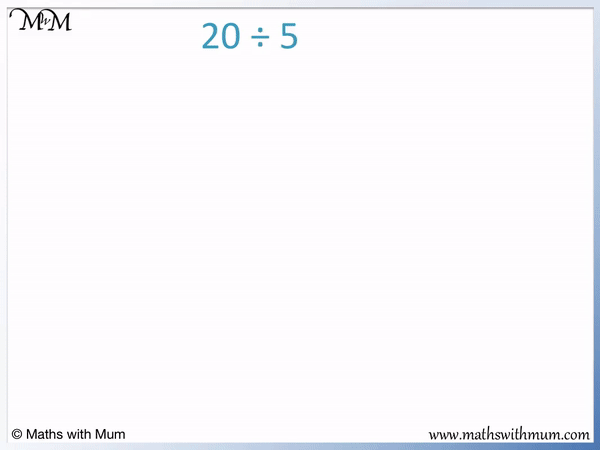We place each of the 20 counters one at a time into the 5 groups.

There are 4 counters in each group and so 20 ÷ 5 = 4.

## Teaching Division by Sharing

Division by sharing is the best way to introduce division to children. This is because this method only involves knowing how to count. The method is also enjoyable and easy to use because it involves physical objects and there are lots of opportunities to use this method in daily life.

When teaching division, it is useful to explain to children what division means.

Division means to share an amount equally. There are lots of opportunities to practise division at home. This can be done with food, toys or counters.

The idea of sharing equally is easy for children to pick up. They will just need to check that each group receives the same amount.

After practising the method using physical objects, division by sharing can be used as a strategy to help with division calculations.

For example, here is 12 ÷ 4.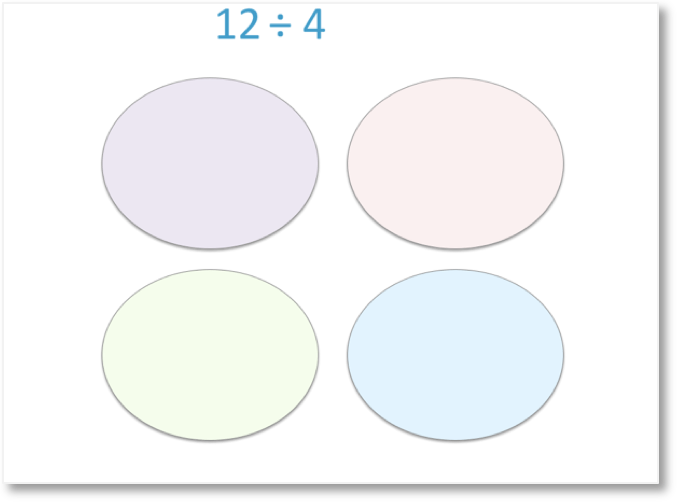Instead of actually using 12 counters and 4 pots, we can draw 4 pots and draw dots for the counters.

We can draw the same number of circles as the number we are dividing by.

We then draw dots in each circle one at a time until we have the total number that we are dividing.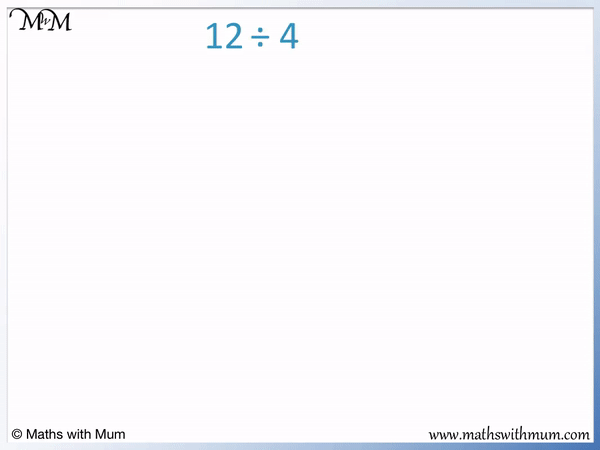We place one dot in each circle one at a time until all 12 have been drawn. We can count the number of dots as we draw them in.

There are 3 dots in each circle and so 12 ÷ 4 = 3.Now try our lesson on Finding Half of a Number where we learn how to halve a number.